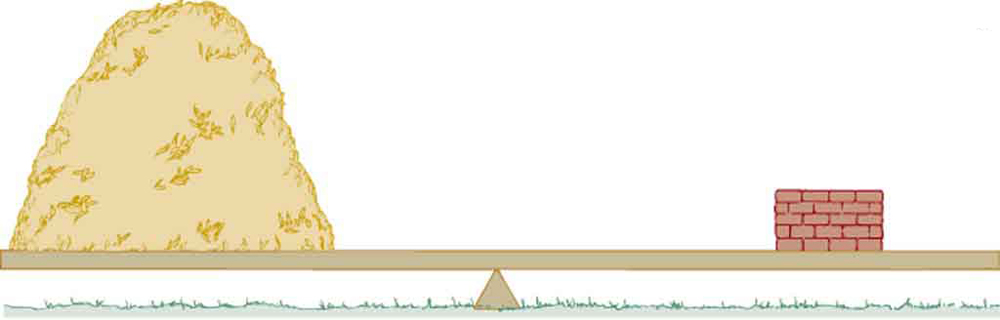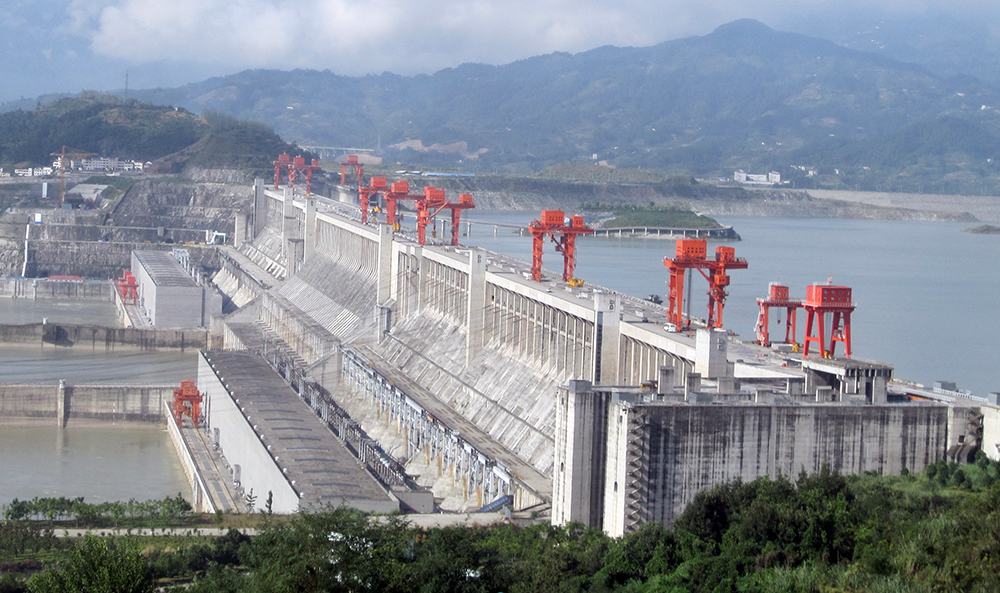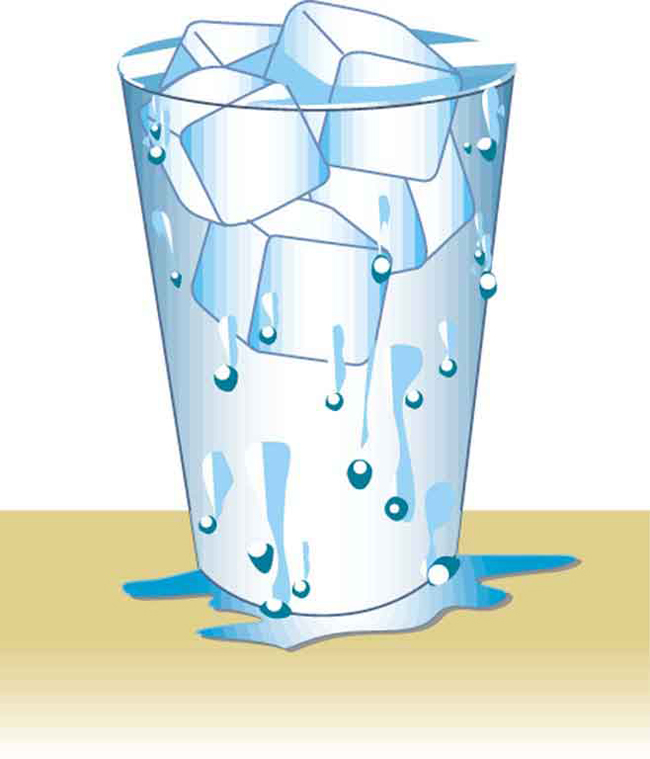Chapter 11 Fluid Statics

# 76 11.2 Density

### Summary

• Define density.
• Calculate the mass of a reservoir from its density.
• Compare and contrast the densities of various substances.

Which weighs more, a ton of feathers or a ton of bricks? This old riddle plays with the distinction between mass and density. A ton is a ton, of course; but bricks have much greater density than feathers, and so we are tempted to think of them as heavier. (See Figure 1.)

Density, as you will see, is an important characteristic of substances. It is crucial, for example, in determining whether an object sinks or floats in a fluid. Density is the mass per unit volume of a substance or object. In equation form, density is defined as

$\boldsymbol{\rho\:=}$$\boldsymbol{\frac{m}{V}},$

where the Greek letter$\boldsymbol{\rho}$(rho) is the symbol for density,$\boldsymbol{m}$is the mass, and$\boldsymbol{V}$is the volume occupied by the substance.

### DENSITY

Density is mass per unit volume.

$\boldsymbol{\rho\:=}$$\boldsymbol{\frac{m}{V}},$

where$\boldsymbol{\rho}$is the symbol for density,$\boldsymbol{m}$is the mass, and$\boldsymbol{V}$is the volume occupied by the substance.

In the riddle regarding the feathers and bricks, the masses are the same, but the volume occupied by the feathers is much greater, since their density is much lower. The SI unit of density is$\boldsymbol{\textbf{kg/m}^3},$representative values are given in Table 1. The metric system was originally devised so that water would have a density of$\boldsymbol{1 \textbf{g/cm}^3},$equivalent to$\boldsymbol{10^3\textbf{ kg/m}^3}.$Thus the basic mass unit, the kilogram, was first devised to be the mass of 1000 mL of water, which has a volume of 1000 cm3.

Substance ρ(103 kg/m3 or g/mL) Substance ρ(103 kg/m3 or g/mL) Substance ρ(103 kg/m3 or g/mL)
Solids Liquids Gases
Aluminum 2.7 Water (4ºC) 1.000 Air $\boldsymbol{1.29\times10^{-3}}$
Brass 8.44 Blood 1.05 Carbon dioxide $\boldsymbol{1.98\times10^{-3}}$
Copper (average) 8.8 Sea water 1.025 Carbon monoxide $\boldsymbol{1.25\times10^{-3}}$
Gold 19.32 Mercury 13.6 Hydrogen $\boldsymbol{0.090\times10^{-3}}$
Iron or steel 7.8 Ethyl alcohol 0.79 Helium $\boldsymbol{0.18\times10^{-3}}$
Lead 11.3 Petrol 0.68 Methane $\boldsymbol{0.72\times10^{-3}}$
Polystyrene 0.10 Glycerin 1.26 Nitrogen $\boldsymbol{1.25\times10^{-3}}$
Tungsten 19.30 Olive oil 0.92 Nitrous oxide $\boldsymbol{1.98\times10^{-3}}$
Uranium 18.70 Oxygen $\boldsymbol{1.43\times10^{-3}}$
Concrete 2.30–3.0 Steam 100º C $\boldsymbol{0.60\times10^{-3}}$
Cork 0.24
Glass, common (average) 2.6
Granite 2.7
Earth’s crust 3.3
Wood 0.3–0.9
Ice (0°C) 0.917
Bone 1.7–2.0
Table 1. Densities of Various SubstancesFigure 1. A ton of feathers and a ton of bricks have the same mass, but the feathers make a much bigger pile because they have a much lower density.

As you can see by examining Table 1, the density of an object may help identify its composition. The density of gold, for example, is about 2.5 times the density of iron, which is about 2.5 times the density of aluminum. Density also reveals something about the phase of the matter and its substructure. Notice that the densities of liquids and solids are roughly comparable, consistent with the fact that their atoms are in close contact. The densities of gases are much less than those of liquids and solids, because the atoms in gases are separated by large amounts of empty space.

### TAKE-HOME EXPERIMENT: SUGAR AND SALT

A pile of sugar and a pile of salt look pretty similar, but which weighs more? If the volumes of both piles are the same, any difference in mass is due to their different densities (including the air space between crystals). Which do you think has the greater density? What values did you find? What method did you use to determine these values?

### Example 1: Calculating the Mass of a Reservoir From Its Volume

A reservoir has a surface area of$\boldsymbol{50.0\textbf{ km}^2}$and an average depth of 40.0 m. What mass of water is held behind the dam? (See Figure 2 for a view of a large reservoir—the Three Gorges Dam site on the Yangtze River in central China.)

Strategy

We can calculate the volume$\boldsymbol{V}$of the reservoir from its dimensions, and find the density of water$\boldsymbol{\rho}$in Table 1. Then the mass$\boldsymbol{m}$can be found from the definition of density

$\boldsymbol{\rho\:=}$$\boldsymbol{\frac{m}{V}}.$

Solution

Solving equation$\boldsymbol{\rho=m/V}$for$\boldsymbol{m}$gives$\boldsymbol{m=\rho{V}}.$

The volume$\boldsymbol{V}$of the reservoir is its surface area$\boldsymbol{A}$times its average depth$\boldsymbol{h}:$

$\begin{array}{lcl} \boldsymbol{V} & \boldsymbol{=} & \boldsymbol{Ah=(50.0\textbf{ km}^2)(40.0\textbf{ m})} \\ {} & \boldsymbol{=} & \boldsymbol{[(50.0\textbf{ km}^2)(\frac{10^3\textbf{ m}}{1\textbf{ km}})^2](40.0\textbf{ m})=2.00\times10^9\textbf{ m}^3} \end{array}$

The density of water$\boldsymbol{\rho}$from Table 1 is$\boldsymbol{1.000\times10^3\textbf{ kg/m}^3}.$Substituting$\boldsymbol{V}$and$\boldsymbol{\rho}$into the expression for mass gives

$\begin{array}{lcl} \boldsymbol{m} & \boldsymbol{=} & \boldsymbol{(1.00\times10^3\textbf{ kg/m}^3)(2.00\times10^9\textbf{ m}^3)} \\ {} & \boldsymbol{=} & \boldsymbol{2.00\times10^{12}\textbf{ kg.}} \end{array}$

Discussion

A large reservoir contains a very large mass of water. In this example, the weight of the water in the reservoir is$\boldsymbol{mg=1.96\times10^{13}\textbf{ N}},$where$\boldsymbol{g}$is the acceleration due to the Earth’s gravity (about$\boldsymbol{9.80\textbf{ m/s}^2}$). It is reasonable to ask whether the dam must supply a force equal to this tremendous weight. The answer is no. As we shall see in the following sections, the force the dam must supply can be much smaller than the weight of the water it holds back.Figure 2. Three Gorges Dam in central China. When completed in 2008, this became the world’s largest hydroelectric plant, generating power equivalent to that generated by 22 average-sized nuclear power plants. The concrete dam is 181 m high and 2.3 km across. The reservoir made by this dam is 660 km long. Over 1 million people were displaced by the creation of the reservoir. (credit: Le Grand Portage)

# Section Summary

• Density is the mass per unit volume of a substance or object. In equation form, density is defined as
$\boldsymbol{\rho\:=}$$\boldsymbol{\frac{m}{V}}.$
• The SI unit of density is$\boldsymbol{\textbf{kg/m}^3}.$

### Conceptual Questions

1: Approximately how does the density of air vary with altitude?

2: Give an example in which density is used to identify the substance composing an object. Would information in addition to average density be needed to identify the substances in an object composed of more than one material?

3: Figure 3 shows a glass of ice water filled to the brim. Will the water overflow when the ice melts? Explain your answer.Figure 3.

### Problems & Exercises

1: Gold is sold by the troy ounce (31.103 g). What is the volume of 1 troy ounce of pure gold?

2: Mercury is commonly supplied in flasks containing 34.5 kg (about 76 lb). What is the volume in liters of this much mercury?

3: (a) What is the mass of a deep breath of air having a volume of 2.00 L? (b) Discuss the effect taking such a breath has on your body’s volume and density.

4: A straightforward method of finding the density of an object is to measure its mass and then measure its volume by submerging it in a graduated cylinder. What is the density of a 240-g rock that displaces$\boldsymbol{89.0\textbf{ cm}^3}$of water? (Note that the accuracy and practical applications of this technique are more limited than a variety of others that are based on Archimedes’ principle.)

5: Suppose you have a coffee mug with a circular cross section and vertical sides (uniform radius). What is its inside radius if it holds 375 g of coffee when filled to a depth of 7.50 cm? Assume coffee has the same density as water.

6: (a) A rectangular gasoline tank can hold 50.0 kg of gasoline when full. What is the depth of the tank if it is 0.500-m wide by 0.900-m long? (b) Discuss whether this gas tank has a reasonable volume for a passenger car.

7: A trash compactor can reduce the volume of its contents to 0.350 their original value. Neglecting the mass of air expelled, by what factor is the density of the rubbish increased?

8: A 2.50-kg steel gasoline can holds 20.0 L of gasoline when full. What is the average density of the full gas can, taking into account the volume occupied by steel as well as by gasoline?

9: What is the density of 18.0-karat gold that is a mixture of 18 parts gold, 5 parts silver, and 1 part copper? (These values are parts by mass, not volume.) Assume that this is a simple mixture having an average density equal to the weighted densities of its constituents.

10: There is relatively little empty space between atoms in solids and liquids, so that the average density of an atom is about the same as matter on a macroscopic scale—approximately$\boldsymbol{10^3\textbf{ kg/m}^3}.$The nucleus of an atom has a radius about$\boldsymbol{10^{-5}}$that of the atom and contains nearly all the mass of the entire atom. (a) What is the approximate density of a nucleus? (b) One remnant of a supernova, called a neutron star, can have the density of a nucleus. What would be the radius of a neutron star with a mass 10 times that of our Sun (the radius of the Sun is$\boldsymbol{7\times10^8\textbf{ m}}$)?

## Glossary

density
the mass per unit volume of a substance or object

### Solutions

Problems & Exercises

1:

$\boldsymbol{1.610\textbf{ cm}^3}$

3:

(a) 2.58 g

(b) The volume of your body increases by the volume of air you inhale. The average density of your body decreases when you take a deep breath, because the density of air is substantially smaller than the average density of the body before you took the deep breath.

4:

$\boldsymbol{2.70\textbf{ g/cm}^3}$

6:

(a) 0.163 m

(b) Equivalent to 19.4 gallons, which is reasonable

8:

$\boldsymbol{7.9\times10^2\textbf{ kg/m}^3}$

9:

$\boldsymbol{15.6\textbf{ g/cm}^3}$

10:

(a)$\boldsymbol{10^{18}\textbf{ kg/m}^3}$

(b)$\boldsymbol{2\times10^4\textbf{ m}}$

## LicenseCollege Physics: OpenStax by OpenStax is licensed under a Creative Commons Attribution 4.0 International License, except where otherwise noted.